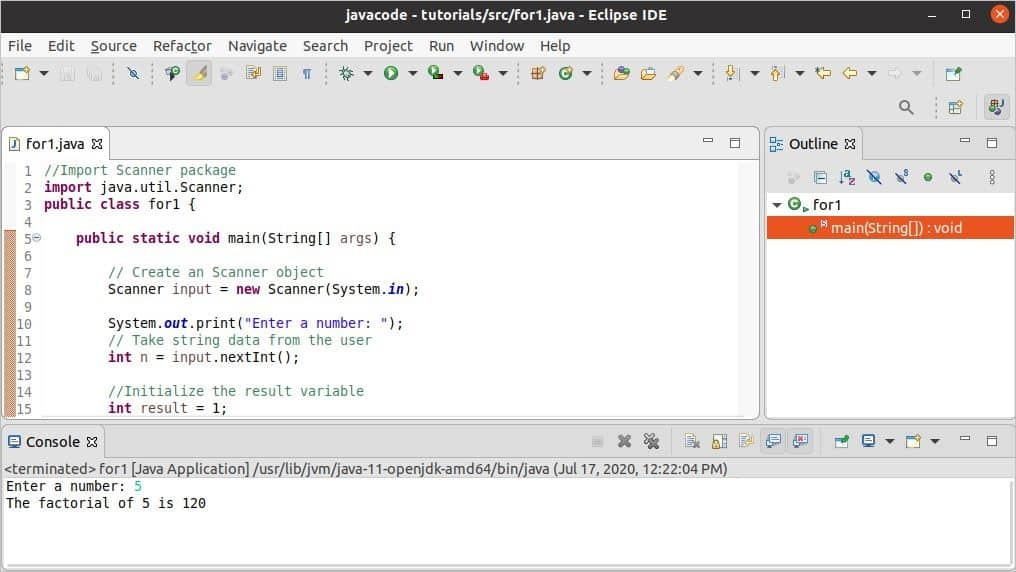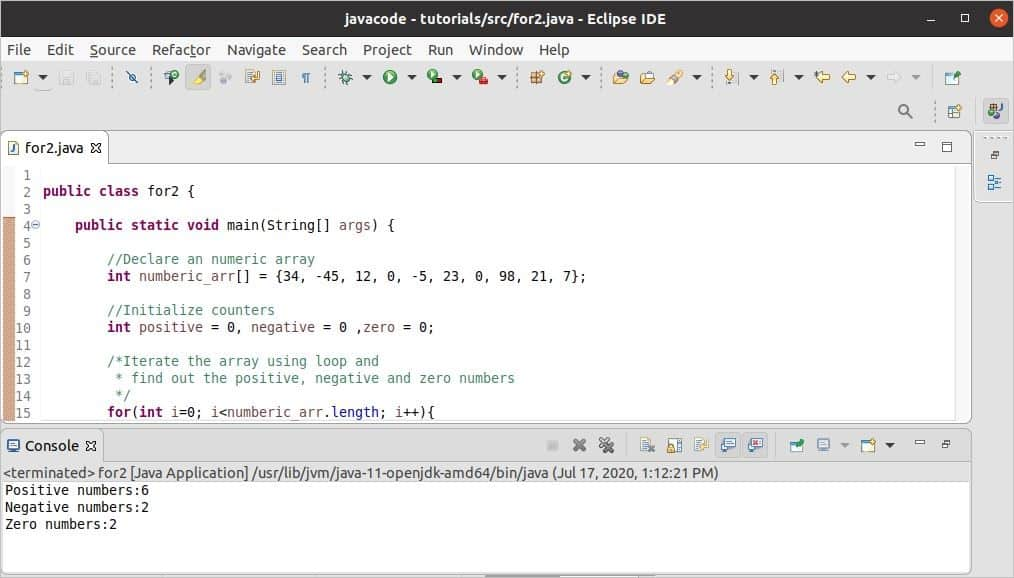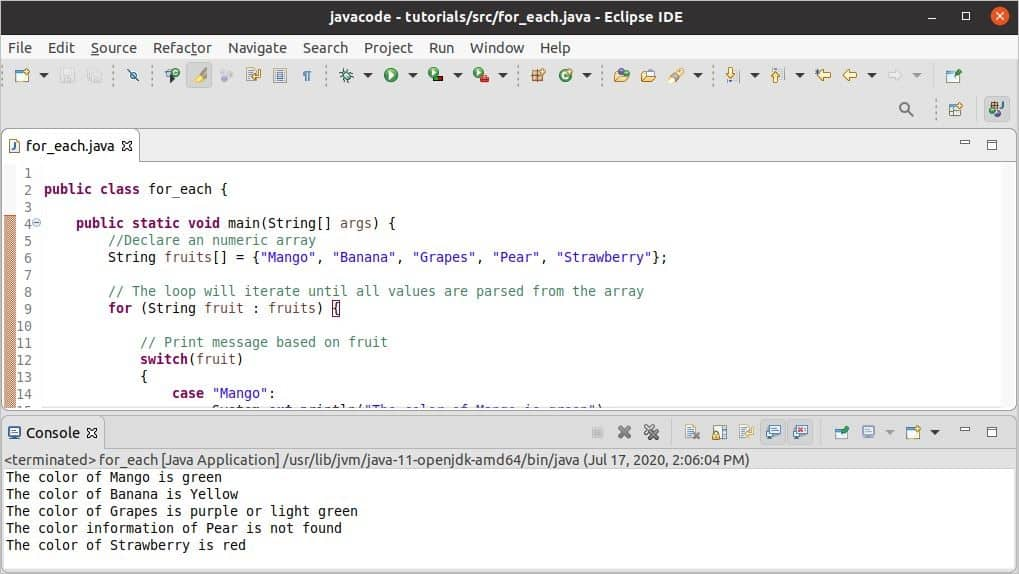Java

# Java for loop

Sometimes, it requires to execute some statements repeatedly for getting any particular output to solve a problem, and this type of task can be done easily by using any type of loop. Generally, three types of loops are supported by most of the programming languages. The ‘for’ loop is one of them. This loop is very useful for doing different types of programming tasks. How ‘for’ loop can be used in Java for multiple purposes is explained in this tutorial.

### Types of ‘for’ loop:

A. ‘for’ loop for a defined number of iteration

Syntax:

for(initialization; termination condition; increment/decrement) {
Statement 1...n
}

This loop has three parts. The first part contains the initialization variable from where the loop will start the iteration. The second part includes the termination condition that is used to terminate the loop. The third part contains an increment or decrement statement based on the initialization variable to reach the termination condition.

B. ‘for’ loop for an undefined number of iteration

Syntax:

for(variable : array or collection) {
Statement 1...n
}

This loop is mainly used for iterating the value from an array or collection variable. This loop will continue the iteration until all items are read.

The different uses of these loops are explained with examples in the next part of this tutorial.

### Example-1: Calculate the factorial of a number

The logical problem can be solved very easily by using a ‘for’ loop. How the factorial value of a number can be calculated by using the ‘for’ loop is shown in this example.  An integer value will be taken from the user and stored in the variable named ‘n’. Another variable, ‘result’ is initialized to 1 to store that value of n!. ‘for’ loop will iterate n times and multiply the value of ‘result’ with the value of ‘i’ in each iteration. The result variable will contain the value of n! after terminating from the loop that will print later.

//Import Scanner package
import java.util.Scanner;
public class for1 {

public static void main(String[] args) {

// Create a Scanner object
Scanner input = new Scanner(System.in);

System.out.print("Enter a number: ");
// Take string data from the user
int n = input.nextInt();

//Initialize the result variable
int result = 1;

/* The loop will iterate for n times
* to calculate the n!
*/

for(int i=1; i<=n; i++) {
// Multiple the value of i with result and store in result
result *= i;
}

// Print the factorial value
System.out.print("The factorial of " + n + " is " + result);

// Close the scanner object
input.close();
}
}

Output:

The following image shows the output of the code. Here, 5 is taken as the value of n and the 5! is 120 that is printed.### Example-2: Count positive, negative and zero numbers from an array

How ‘for’ loop can be used to read the values of a numeric array and count the total positive, negative, and zero numbers in the array are shown in this example. A numeric array of 10 elements is defined in the code. The length property is used in Java to count the total number of elements of an array object. It is used in the ‘for’ loop to define the number of times the loop will iterate. Positive, negative, and zero counter variables are initialized to 0 for storing the result. Next, the ‘for’ loop is used to read each value of the array and increment the particular counter value by checking ‘if’ condition. All counter variables are printed later.

public class for2 {

public static void main(String[] args) {

//Declare an numeric array
int numberic_arr[] = {34, -45, 12, 0, -5, 23, 0, 98, 21, 7};

//Initialize counters
int positive = 0, negative = 0 ,zero = 0;

/*Iterate the array using loop and
* find out the positive, negative and zero numbers
*/

for(int i=0; i 0)
{
positive++;
}
else if (numberic_arr[i] < 0)
{
negative++;
}
else
{
zero++;
}
}

//Print the counter values
System.out.print("Positive numbers:" + positive + "\n" +
"Negative numbers:" + negative + "\n" +
"Zero numbers:" + zero);
}
}

Output:

The following image shows the output of the code. There are 6 positive, 2 negative, and 2 zero numbers in the array.### Example-3: Print a particular message based on each value of an array

How many times the ‘for’ loop will iterate is defined in the previous two examples.  In this example, the ‘for’ loop is used with a ‘switch-case’ statement to print a particular message based on each value of an array. How many times this loop will iterate depends on the number of elements of the array. So, the loop will iterate until all elements of the array are parsed.  In each iteration, it will match the element value with the case value and print the particular message where the match found; otherwise, it will print the message defined in the default section.

public class for_each {

public static void main(String[] args) {
//Declare an numeric array
String fruits[] = {"Mango", "Banana", "Grapes", "Pear", "Strawberry"};

// The loop will iterate until all values are parsed from the array
for (String fruit : fruits) {

// Print message based on fruit
switch(fruit)
{
case "Mango":
System.out.println("The color of Mango is green");
break;

case "Banana":
System.out.println("The color of Banana is Yellow");
break;

case "Grapes":
System.out.println("The color of Grapes is purple or light green");
break;

case "Strawberry":
System.out.println("The color of Strawberry is red");
break;

default:
System.out.println("The color information of " + fruit +
}
}
}
}

Output:

The following image shows the output of the code.  Here, no match is found for the ‘’Pear,” and the default message is printed for this value only.### Conclusion:

‘for’ loop is very useful for solving many types of problems easily. Iterating loop for the fixed number of times and reading data from array objects are explained in this tutorial by using various examples. I hope this tutorial will help the new Java users to apply ‘for’ loop in their code properly.